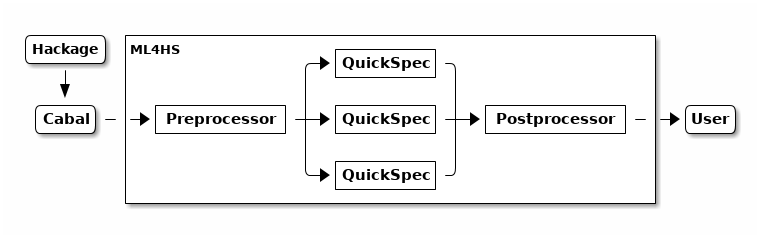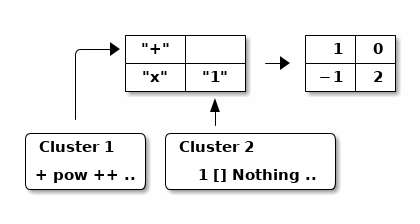# Scaling Automated Theory Exploration in Haskell

 Chris Warburton Katya Komendantskaya cmwarburton@dundee.ac.uk e.komendantskaya@dundee.ac.uk University of Dundee AI4FM 2015

# Theory Exploration

$(\Sigma, V) \overset{TE}{\rightarrow} \text{Terms}(\Sigma, V)$

Theory Exploration describes any system for turning a "theory" (signature and variables) into terms of that theory which are well-formed, provable and interesting.

## Comparison Criteria

• How do we generate terms?
• How do we guarantee well-formedness?
• How do we prove terms?
• What is considered "interesting"?

# (Interactive) Theory Exploration

Mathematical theory exploration proceeds by applying, under the guidance of a human user, various algorithmic reasoners for producing new formulae from given ones and aims at building up (large) mathematical knowledge bases in an efficient, reliable, well-structured, re-usable, and flexible way. -- (Buchberger 2004)

Example: Theorema

• How do we generate terms? User-driven
• How do we guarantee well-formedness? Types and pre-conditions
• How do we prove terms? Automated Theorem Provers
• What is considered "interesting"? User-driven

# Automated Theory Exploration

MathematicalAutomated theory exploration proceeds by applying, under the guidance of a human user, various algorithmic reasoners for producing new formulae from given ones and aims at building up (large) mathematical knowledge bases in an efficient, reliable, well-structured, re-usable, and flexible way.

Examples: IsaCoSy, Hipster, HipSpec

• How do we generate terms? Propose equations between enumerated expressions
• How do we guarantee well-formedness? Types
• How do we prove terms? Automated Theorem Provers
• What is considered "interesting"? Un-derivable from previous equations

# Why Automated Theory Exploration?

Strengths:

• Exposing problematic assumptions
• Finding simple relationships
• Autonomous
• Open-ended, anytime

Weaknesses:

• Scales poorly with theory & formula size
• Can't propose new notions/definitions

Problem: Doesn't seem to align very well with general Mathematics

# Why Automated Theory Exploration?

Strengths:

• Exposing problematic assumptions
• Finding simple relationships
• Autonomous
• Open-ended, anytime

Weaknesses:

• Scales poorly with theory & formula size
• Can't propose novel notions/axioms

Solution: Aligns well with programming

• Equational: definitions, rewrite rules & coercions
• Pure: Effects are separate from computation
• Strong types: Algebraic datatypes, parametricity
• Popular: Relatively large code repositories (eg. Hackage)

Haskell code often follows algebraic laws which Haskell is unable to express!

# Identifying Improvements

HipSpec is state-of-the-art. How might it be scaled up?

• Well-formedness: Types work well enough
• Proof: ATP works well enough

# Improving Term Generation

• In theory: enumeration scales exponentially
• In reality: types constrain the combinations
• Requires investigation!
• Search problem:
• Well-studied in Artificial Intelligence
• Heuristics, optimisation
• Machine Learning

# Improving "Interestingness"

• Un-derivability is elegant, but misses some intuitions
• "Non-obvious" reductions
• Irrelevant/incidental relationships
• Well-studied in Machine Learning and Data Mining
• e.g. mutual information, "surprise"
• What kinds of properties do we tend to care about?
• Look at what we tend to test!

# Current Work: ML4HS• Framework for ATE experiments in Haskell
• Based around QuickCheck (HipSpec's exploration component)
• Divide and conquer

# Generating Theories

• Use Cabal to get/build package
• Use GHC plugin to extract top-level names and ASTs
• Try to put each name in a theory
• Non-exported names
• Artifacts of Core (eg. typeclass dictionaries)
• Type errors
• Generate Haskell project with the successes
• Use Nix to handle dependencies

# Divide and Conquer

• Inspired by premise selection in ATP clustering in ML4PG
• No need to compare everything to everything else
• Select sub-sets which appear related
• Initial approach: cluster by similarity
• Future approaches:
• Pair-wise premise selection?
• Off-the-shelf ML algorithms (eg. ANN)?

# Clustering

• Extract definitions (Core), via GHC plugin
• Topological sort by dependencies
• Feature extraction by recurrent clustering:
• Turn AST into a matrix
• Replace symbols by their cluster number
• Repeat

# Recurrent Clustering Example

inc x = x + 1# Applications

• Finding redundancy/duplication across libraries
• Refactoring aid
• Finding isomorphisms
• Finding rewrite rules
• Finding new typeclasses (sets of "laws")

# Acknowlegements

Thank you to the HipSpec team at Chalmers University (Moa Johansson, Koen Claessen, Nick Smallbone, Dan Rosén and Irene Lobo Valbuena) for useful discussions of these ideas, and Ouanis Seddaoui for help with our implementation.